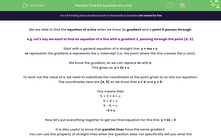# Find the Equation of a Line

In this worksheet, students will find the equations of straight lines when given the line's gradient and a point it passes through, or these can be calculated. They will also apply the similar properties of parallel lines.Key stage:  KS 4

Year:  GCSE

GCSE Subjects:   Maths

GCSE Boards:   Pearson Edexcel, OCR, Eduqas, AQA,

Curriculum topic:   Algebra, Graphs of Equations and Functions

Curriculum subtopic:   Graphs Straight Line Graphs

Difficulty level:#### Worksheet Overview

We are able to find the equation of a line when we know its gradient and a point it passes through.

e.g. Let's say we want to find an equation of a line with a gradient 2, passing through the point (4, 5).

Start with a general equation of a straight line: y = mx + c

m represents the gradient, c represents the y-intercept (i.e. the point where the line crosses the y-axis).

We know the gradient, so we can replace m with 2.

This gives us: y = 2x + c

To work out the value of c, we need to substitute the coordinates of the point given to us into our equation.

The coordinates here are (4, 5) so we know that x = 4 and y = 5.

This means that:

5 = 2 × 4 + c

5 = 8 + c

5 - 8 = c

-3 = c

Now let's put everything together to get our final equation for this line: y = 2x - 3

It is also useful to know that parallel lines have the same gradient.

You can use this property of straight lines when the question does not specifically tell you what the gradient is.

In this activity, we will find the equations of straight lines when we are given or can calculate, the line's gradient and a point it passes through.

You will also need to recognise and apply the similar properties of parallel lines.

Want a bit more help with this before you begin? Why not watch this short video?

### What is EdPlace?

We're your National Curriculum aligned online education content provider helping each child succeed in English, maths and science from year 1 to GCSE. With an EdPlace account you’ll be able to track and measure progress, helping each child achieve their best. We build confidence and attainment by personalising each child’s learning at a level that suits them.

Get started